#### Vol. 32, No. 1, 1970

 Download this articleFor screen For printingRecent Issues Vol. 323: 1  2 Vol. 322: 1  2 Vol. 321: 1  2 Vol. 320: 1  2 Vol. 319: 1  2 Vol. 318: 1  2 Vol. 317: 1  2 Vol. 316: 1  2Online Archive Volume: Issue:The Journal Subscriptions Editorial Board Officers Contacts Submission Guidelines Submission Form Policies for Authors ISSN: 1945-5844 (e-only) ISSN: 0030-8730 (print) Special Issues Author Index To Appear Other MSP Journals
On an initial value problem in the theory of two-dimensional transonic flow patterns

### Stefan Bergman

Vol. 32 (1970), No. 1, 29–46
##### Abstract

In the case of the differential equation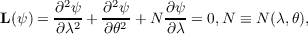where N is an analytic function, the integral operator of the first kind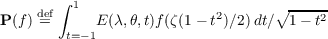transforms analytic functions of a complex variable ζ = λ + i𝜃 into solutions of L(ψ) = 0. Here E is a fixed function which depends only on L, while f(ζ) is an arbitrary analytic function of the complex variable ζ;f is assumed to be regular at ζ = 0. Using this operator, one shows that many theorems valid for analytic functions of the complex variable can be generalized for the solutions ψ of L(ψ) = 0. Continuing ψ(λ,𝜃) to complex values U = λ + iΛ and setting λ = 0, one shows that many theorems in the theorems in the theory of functions of a real variable can be generalized to the case of solutions of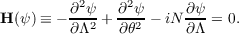By change of the variables,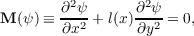l(x) > 0 for x < 0,l(x) < 0 for x > 0,l(O) = 0, when considered for x < 0 can be reduced to the equation L(ψ) = 0. The variables can be chosen so that U = 0 corresponds to x = 0. However, in this case the function N(λ) becomes singular at λ = 0. Nevertheless, one can apply the theory of the so-called integral operators of the second kind. If ψ(0,𝜃) = χ1(𝜃) and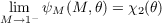are given, one can determine the function f. Here M is the Mach number. In this way one can determine from χ1 and χ2 the location and character of singularities of ψ in the subsonic region. When considering ψ in the supersonic region, one can show that some theorems on functions of one real variable can be generalized to the case of certain sets of particular solutions ψν,𝜃)= 1,2, , of H(ψ) = 0.

Primary: 76.35
##### Milestones